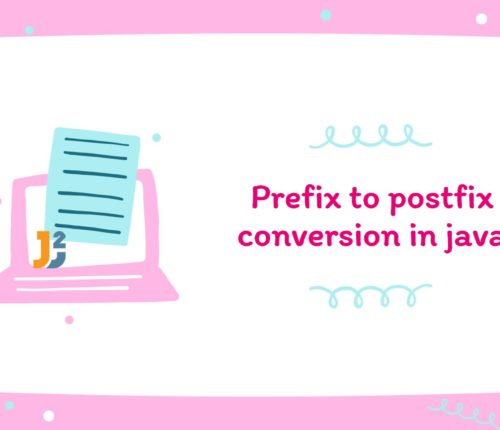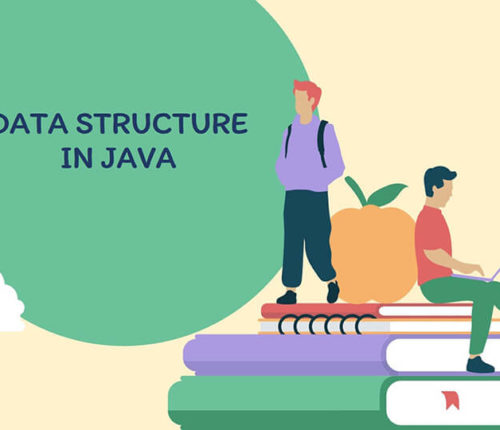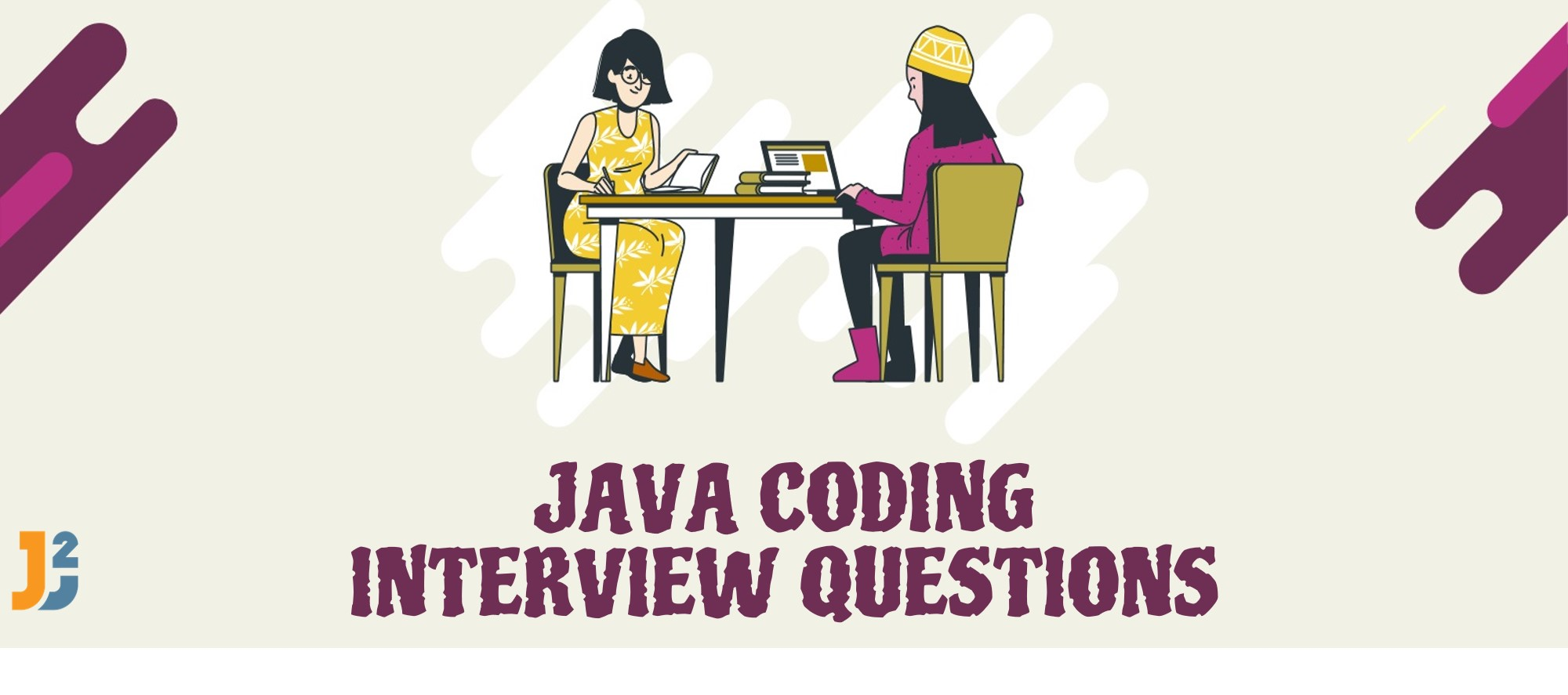# Check if it is possible to reach end of given Array by Jumping

If you want to practice data structure and algorithm programs, you can go through Java coding interview questions.

﻿

﻿

## Problem

Given an array with positive integers as elements indicating the maximum length of a jump which can be made from any position in the array. Check if it is possible to have a jumps combination so that you can eventually reach the end of given array. Print "true" if it is possible, otherwise, print "false".

Input :
[1, 5, 2, 1, 0, 2, 0]

Output :
true

Input :
[5, 4, 3, 2, 1, 0, 0]

Output :
false

## Solution

APPROACH – I (RECURSIVE)

This a very basic brute force approach in which we make all the possible jumps from any current position.
We start with the current position at index zero in the array, and make a recursive call by changing the current position by adding all the possible jump lengths one by one. We also need to make sure that we stay in bounds of the given array, that is why we only make the jump if the current position after adding this jump length stays in bound.

Positive Base case : If the current position is equal to array.length, this means that we have successfully reached the end of the given array, that is, a jumps combination exists with which we can reach end of given array, hence we return true from here.

Negative base case : if maximum length of any jump possible from any current position is equal to zero, this means that there is no way possible with which we can reach the end of the given array as we cannot move even a single position from our current position.

Time complexity Discussion :

As from every point we are making all the possible jumps and in worst case from any position, we can make ‘n’ jumps and there are n possible spots from where we can make these jumps, therefore, worst time complexity of this algorithm for solving this problem is O(n^n), where ‘n’ is the length of the given array.

APPROACH – II (Iterative) :

In the previous approach we can clearly see so many duplications of recursive call which find the result of same position again and again, therefore, we can use Dynamic Programming to solve the given problem and also reduce the running time complexity of the algorithm.

In this Dynamic Programming approach, we calculate result for every index that is, if it is possible to reach to that ‘i’th index from 0th index with a combination of possible jumps.

Therefore, we make a boolean DP array for storing the result for every index which is false initially except the first index as this is where we start the journey from.

• Some Points which are needed to be considered are :
(i) We can make further jumps from any position only if that position itself is reachable from Zeroth index.
(ii) We can not move any further from an index if the maximum allowed length of any jump is Zero.
Therefore, we can only move from any position only if that position itself is reachable and length of jump allowed from that position is not zero.

Our main motive is to find if there exists a path or jump combination from Zeroth index to Last index.

• To prove this, we can agree on the fact that, If there exist a path or jump combination from Zeroth index to any Intermediate Index, and also there exist a path or jump combination from Intermediate index to Last Index, Then the the last index will surely be reachable from Zeroth index.

Time complexity Discussion :
As in this approach also we are making jumps of all possible lengths which can potentially be ‘n’ in worst case, and we are doing this in loop for every index of the array, then, the worst time complexity of this algorithm for solving this problem is O(n^2), where ‘n’ is length of the given array.

That’s all about to check if it is possible to reach end of given Array

import_contacts

### You may also like:## Related Posts

•30 April

### Convert Postfix to Infix in Java

Learn about how to convert Postfix to Infix in java.

•30 April

### Convert Prefix to Postfix in Java

Learn about how to convert Prefix to Postfix in java.

•16 April

### Data Structures in java

In this post, we will see about various data structures in java. Data structure is a way of storing and organizing data. Data structure provide a way to process and store data efficiently. For example: Imagine you have pile of books on the table and you are going to read these books one by one […]

•29 November

### Top 100+ Java coding interview questions

I have been posting data structure and coding interview questions on various topics such as Array, Queue, Stack, Binary tree, LinkedList, String, Number, ArrayList, etc. So I am consolidating a list of java coding interview questions to create an index post. I will keep adding links to this post whenever I will add new java […]

•18 April

### Minimum Number of Jumps to reach last Index

If you want to practice data structure and algorithm programs, you can go through Java coding interview questions. In this post, we will see how to find Minimum Number of Jumps to reach last Index. Problem Given an array A of positive integers possibly zeroes, every index indicating the maximum length of a jump that can […]

•28 March

### Sort an array of 0s, 1s and 2s

If you want to practice data structure and algorithm programs, you can go through Java coding interview questions. In this post, we will see how to sort an array of 0s, 1s and 2s.We have already seen a post on sort 0s and 1s in an array. Problem Given an array containing zeroes, ones and twos […]

## Subscribe to our newletter

Get quality tutorials to your inbox. Subscribe now.